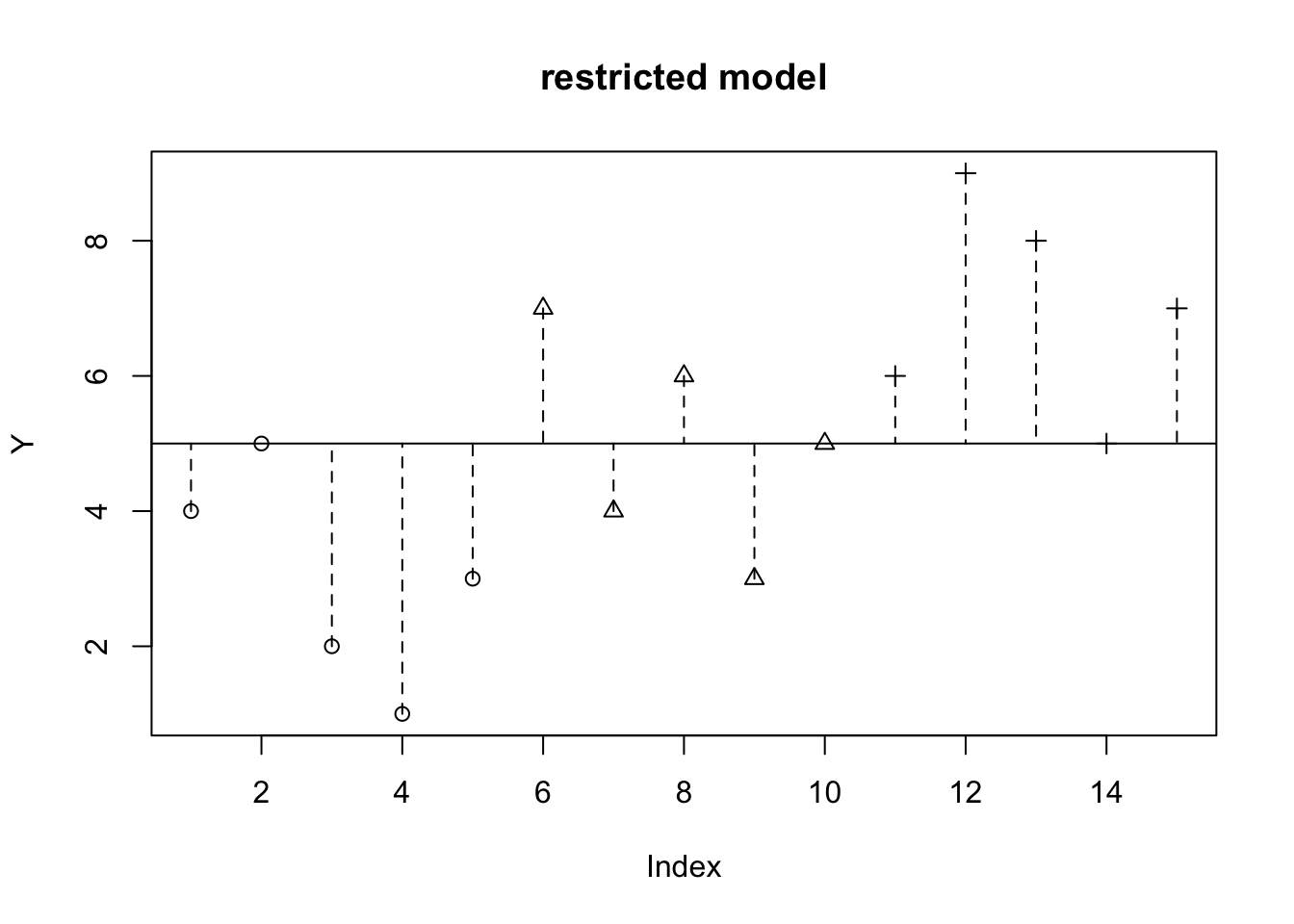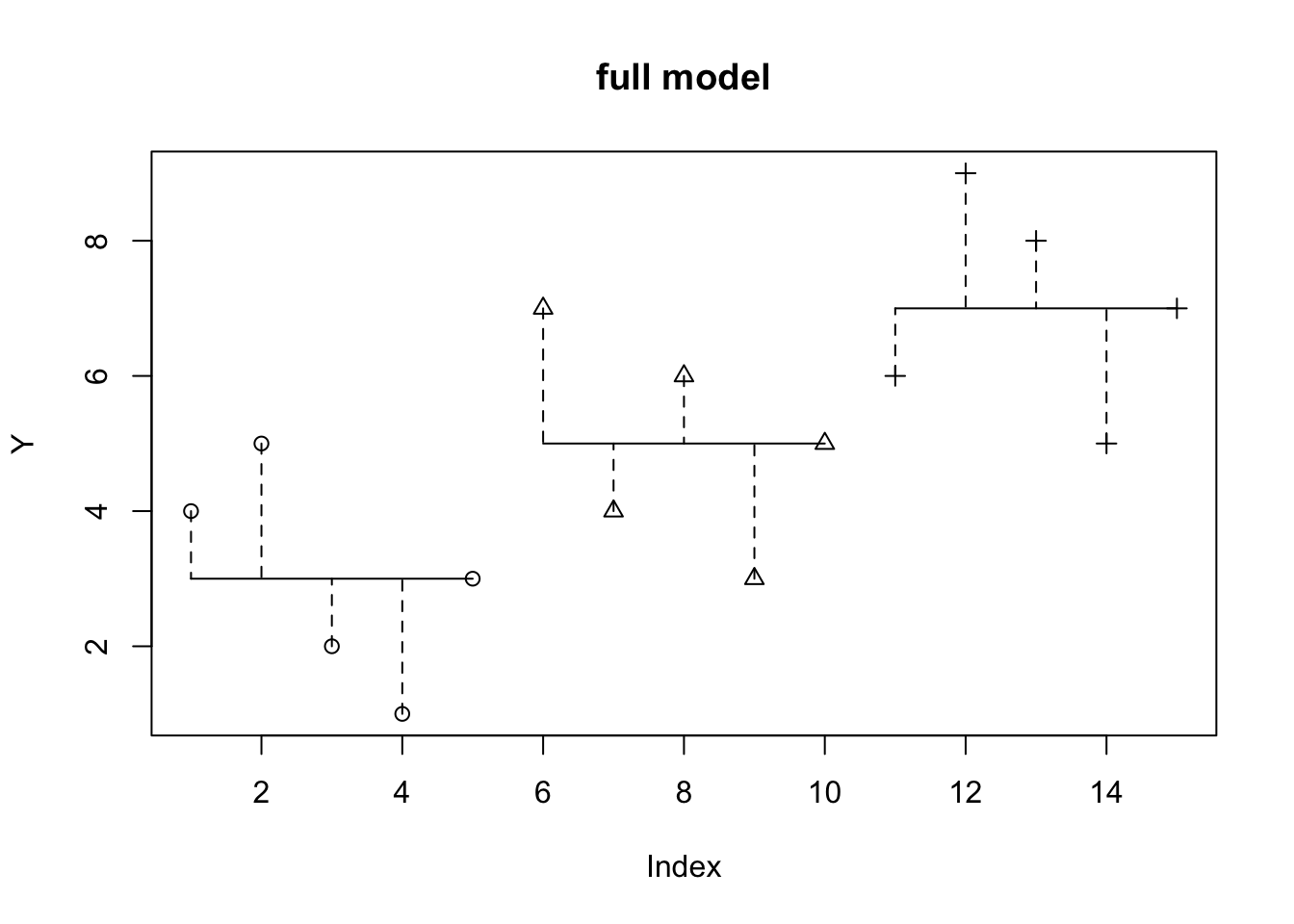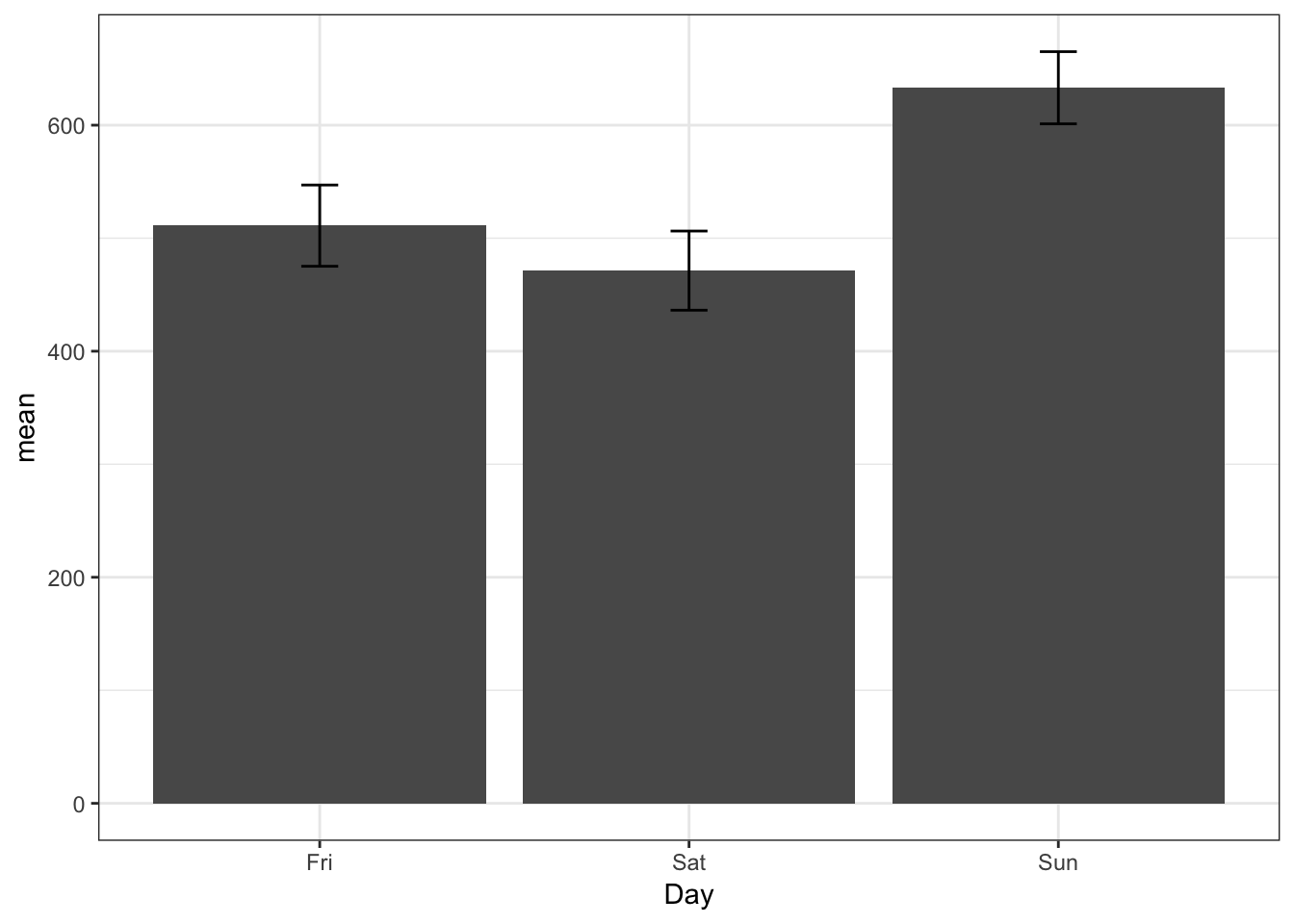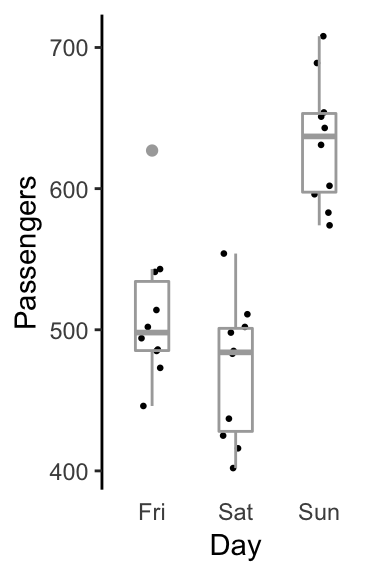## One-way between subjects ANOVA

Assume we have collected data from 15 subjects, each of whom were randomly assigned to one of three groups:

The single factor between subjects analysis of variance (ANOVA) tests the null hypothesis that the means of the populations from which the three samples were drawn, are the same.

One way of thinking about the ANOVA is that it partitions the total variance in the dependent variable into two parts: between-groups variance (variance due to differences between groups) and within-group variance (the variability within a group). The F-test is then a test of whether the between-groups variance is significantly greater than the within-groups variance—in other words, are the observed differences larger than what one would expect given the typical variability within a group?

The other way of thinking about the ANOVA is using a model comparison approach. Under a restricted model, one seeks to account for the dependent variable using a single parameter—the grand mean. Under a full model, one introduces additional parameters allowing one to adjust the value of the dependent variable depending on group membership:

$\begin{eqnarray} m_{restricted}: &Y_{ij} &= \mu + \epsilon_{ij} \\ m_{full}: &Y_{ij} &= \mu + \alpha_{j} + \epsilon_{ij} \end{eqnarray}$

Which model fits the data better? Of course, the full model will fit the data better, as it has more parameters (and hence more flexibility). The real question is, whether the increase in model fit (or the decrease in model error, or residual), is worth giving up the degrees of freedom inherent in having to estimate additional parameters? This is the question that the F-test in the ANOVA answers.

In the above example, the restricted model postulates that the data can be fit using a single parameter, the grand mean $$\mu$$. The full model postulates that the data should be fit using three parameters, $$\mu_{1}$$, $$\mu_{2}$$ and $$\mu_{3}$$—i.e. a different mean for each group.

We can represent these two models graphically. The restricted model assumes all the data are fit by a single parameter, the grand mean $$\mu$$ (The vertical dashed lines indicate the model prediction errors):

Y <- c(4,5,2,1,3,7,4,6,3,5,6,9,8,5,7)
myFac <- c(1,1,1,1,1,2,2,2,2,2,3,3,3,3,3)
plot(Y, pch=myFac, main="restricted model")
abline(h=mean(Y))
for (i in 1:length(Y)) {
lines(c(i,i), c(Y[i], mean(Y)), lty=2)
}Under the full model, we estimate a different mean for each group:

Y <- c(4,5,2,1,3,7,4,6,3,5,6,9,8,5,7)
myFac <- c(1,1,1,1,1,2,2,2,2,2,3,3,3,3,3)
plot(Y, pch=myFac, main="full model")
for (j in 1:3) {
w <- which(myFac==j)
lines(c(min(w),max(w)),c(mean(Y[w]),mean(Y[w])))
for (i in 1:length(w)) {
lines(c(w[i],w[i]), c(Y[w[i]], mean(Y[w])), lty=2)
}
}Again, the dashed vertical lines indicate model error. Obviously the full model predicts the data better. The question ANOVA will answer is, whether the increase in model fit (the decrease in prediction error) is worth giving up the degrees of freedom necessary to estimate the additional parameters.

In R it’s very simple to perform an ANOVA using the aov() function:

m1 <- aov(Y ~ factor(myFac))
summary(m1)
##               Df Sum Sq Mean Sq F value Pr(>F)
## factor(myFac)  2     40    20.0       8 0.0062 **
## Residuals     12     30     2.5
## ---
## Signif. codes:  0 '***' 0.001 '**' 0.01 '*' 0.05 '.' 0.1 ' ' 1

In this case the main effect of myFac is significant at p=0.006196, so we would reject the null hypothesis that the three groups were sampled from the same population (or populations with the same mean).

Another way of running the anova that highlights the fact that we are fitting three parameters, is to use the lm() function:

m2 <- lm(Y ~ factor(myFac))
summary(m2)
##
## Call:
## lm(formula = Y ~ factor(myFac))
##
## Residuals:
##    Min     1Q Median     3Q    Max
##     -2     -1      0      1      2
##
## Coefficients:
##                Estimate Std. Error t value Pr(>|t|)
## (Intercept)      3.0000     0.7071   4.243  0.00114 **
## factor(myFac)2   2.0000     1.0000   2.000  0.06866 .
## factor(myFac)3   4.0000     1.0000   4.000  0.00176 **
## ---
## Signif. codes:  0 '***' 0.001 '**' 0.01 '*' 0.05 '.' 0.1 ' ' 1
##
## Residual standard error: 1.581 on 12 degrees of freedom
## Multiple R-squared:  0.5714, Adjusted R-squared:    0.5
## F-statistic:     8 on 2 and 12 DF,  p-value: 0.006196

The parameter estimates are called Coefficients and are listed in the column marked Estimate. In this case the estimate for the first group (called Intercept in the anova output) is 3.0000. The estimate for the mean of group two is equal to the Intercept plus 2.0000, which equals 5.0000. Likewise the estimate for group three is 3.0000 + 4.0000 which equals 7.0000.

We can then perform an F-test by applying the anova() command to the model object m2:

anova(m2)
## Analysis of Variance Table
##
## Response: Y
##               Df Sum Sq Mean Sq F value   Pr(>F)
## factor(myFac)  2     40    20.0       8 0.006196 **
## Residuals     12     30     2.5
## ---
## Signif. codes:  0 '***' 0.001 '**' 0.01 '*' 0.05 '.' 0.1 ' ' 1

The F-test of the main effect of the factor is called an omnibus test. A significant test indicates only that the population means are not equal—we would need to perform follow-up tests to find out specifically which groups differ. This topic will be covered in the next chapter.

### Testing Assumptions

Two testable assumptions of ANOVA are homogeneity of variances (the variances in each group are the same) and normality (the data within each group are normally distributed).

#### Normality

To test the normality assumption we can use the Shapiro-Wilk normality test. In R the function is shapiro.test().

Let’s load some sample data (numbers of passengers on a ferry for 10 weeks measured on each Friday, Saturday and Sunday). We will use the summary() R function to get an initial look at the data, and then the aggregate() function to compute the means and standard deviations across days:

ferrydata=read.table("../data/ferrydata.csv", header=T, sep=",")
summary(ferrydata)
##    Passengers     Day
##  Min.   :402.0   Fri:10
##  1st Qu.:485.0   Sat:10
##  Median :512.5   Sun:10
##  Mean   :538.5
##  3rd Qu.:600.5
##  Max.   :708.0
aggregate(list(Mean_Passengers=ferrydata$Passengers), list(Day=ferrydata$Day), mean)
##   Day Mean_Passengers
## 1 Fri           511.1
## 2 Sat           471.3
## 3 Sun           633.1
aggregate(list(SD_Passengers=ferrydata$Passengers), list(Day=ferrydata$Day), sd)
##   Day SD_Passengers
## 1 Fri      50.25369
## 2 Sat      48.96268
## 3 Sun      44.64788
shapiro.test(subset(ferrydata$Passengers, ferrydata$Day=="Fri"))
##
##  Shapiro-Wilk normality test
##
## data:  subset(ferrydata$Passengers, ferrydata$Day == "Fri")
## W = 0.89508, p-value = 0.1933
shapiro.test(subset(ferrydata$Passengers, ferrydata$Day=="Sat"))
##
##  Shapiro-Wilk normality test
##
## data:  subset(ferrydata$Passengers, ferrydata$Day == "Sat")
## W = 0.94157, p-value = 0.5706
shapiro.test(subset(ferrydata$Passengers, ferrydata$Day=="Sun"))
##
##  Shapiro-Wilk normality test
##
## data:  subset(ferrydata$Passengers, ferrydata$Day == "Sun")
## W = 0.95184, p-value = 0.6903

ANOVA is generally robust to violations of normality, as long as all groups violate from normality in the same way, and as long as the number of observations in each group is the same.

If there is a violation of the normality assumption and you are concerned about inflated Type-I error rates, one approach is to apply a transformation to the data to make it normal. Some common transformations include square-root, logarithm, reciprocal, inverse-sine (see text). The tradeoff is that although these mathematical transformations may fix non-normality, you must keep in mind that conclusions based on transformed data only apply to the transformed data, not necessarily to the original data. This can make interpretation difficult.

#### Homogeneity of Variances

To test homogeneity of variances we can use the bartlett test, in R the function is bartlett.test():

ferrydata=read.table("../data/ferrydata.csv", header=T, sep=",")
bartlett.test(Passengers ~ Day, data=ferrydata)
##
##  Bartlett test of homogeneity of variances
##
## data:  Passengers by Day
## Bartlett's K-squared = 0.1298, df = 2, p-value = 0.9372

If there is a violation of homogeneity of variance, then one approach is to use the Welch correction (as described in your text), which adjusts the degrees of freedom to compensate for the unequal variances. In R you can do this using the oneway.test() function.

ANOVA is generally robust to violations of homogeneity of variances, as long as sample sizes are equal, and as long as the normality assumption holds.

## Graphics

There are many ways to plot your data. Some common plotting functions that are included in the base distribution of R are:

• plot()
• boxplot()
• barplot()

I suggest you look at the R help files for each function, and the example code at the bottom of each help file, to see how these work.

There is a more powerful graphics package you can add to R called ggplot2. To download and install it into R issue the following command in R:

install.packages("ggplot2")

Then each time you launch R and you wish to use the package type:

library(ggplot2)

There is lots of documentation about the ggplot2 package online, I suggest doing a google search.

The ggplot2 package is also included in the meta-package called tidyverse, which includes other useful packages like dplyr and others.

The following new (as of 2017) book is a great introduction to ggplot2, dplyr and other basic R functionality:

R for Data Science by Hadley Wickham & Garrett Grolemund. O’Reilly (2017) ISBN: 978-1491910399

The book has a website where you can read everything online:

Here are some sample plots

First calculate some summary statistics from our data

library(dplyr)
##
## Attaching package: 'dplyr'
## The following objects are masked from 'package:stats':
##
##     filter, lag
## The following objects are masked from 'package:base':
##
##     intersect, setdiff, setequal, union
ferrysummary <- ferrydata %>% group_by(Day) %>% summarise(n = length(Passengers),
n = n(),
mean = mean(Passengers),
sd = sd(Passengers),
se = sd(Passengers)/sqrt(n),
ci95 = qt(0.975, df=n-1) * se)
library(ggplot2)
ggplot(data=ferrysummary, aes(x=Day, y=mean)) +
geom_bar(stat="identity", position=position_dodge()) +
geom_errorbar(aes(ymin=mean-ci95, ymax=mean+ci95, width=0.1)) +
theme_bw()Another style

ggplot(data=ferrydata, mapping=aes(x=Day, y=Passengers)) +
geom_point(position=position_jitter(width=0.15, height=0), color="black", size=0.5) +
geom_boxplot(fill=NA, width=0.4, size=0.5, color="DarkGray") +
theme_classic() +
theme(axis.line.x=element_blank(), axis.ticks.x=element_blank()) +
labs(x="Day", y="Passengers")Boxplot with raw data superimposed.

ggsave("ferry_fig.pdf", width=3, height=6, units="in")